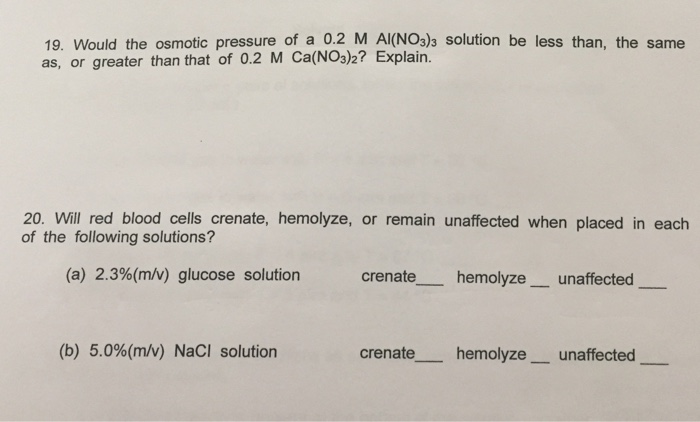# Question & Answer: Would the osmotic pressure of a 0.2 M Al(NO_3)_3 solution be less than, the same as, or…..

Detailed explanations for both questions would be helpful, thank you!Would the osmotic pressure of a 0.2 M Al(NO_3)_3 solution be less than, the same as, or greater than that of 0.2 M Ca(NO_3)_2? Explain. Will red blood cells crenate, hemolyze, or remain unaffected when placed in each of the following solutions? (a) 2.3%(m/v) glucose solution crenate _____ hemolyze _____ unaffected _____ (b) 5.0%(m/v) NaCl solution crenate _____ hemolyze _____ unaffected _____

Question 19.

First, let us define the osmotic pressure:

Don't use plagiarized sources. Get Your Custom Essay on
Question & Answer: Would the osmotic pressure of a 0.2 M Al(NO_3)_3 solution be less than, the same as, or…..
GET AN ESSAY WRITTEN FOR YOU FROM AS LOW AS \$13/PAGE

Posm = i*M*RT

i = number of ions in solution, Molarity of species, R = ideal gas constant, T temperature

now,

ions of Al(NO3)3 = Al3+ + 3NO3- = 1+3 = 4 ions

ionf of Ca(NO3)2 = Ca+2 + 2NO3- = 1+2 = 3 ions

therefore,

i of Al(NO3)3 > i of Ca(NO3)2

then

the osmotic pressure of Al(NO3)3 must be GREATER